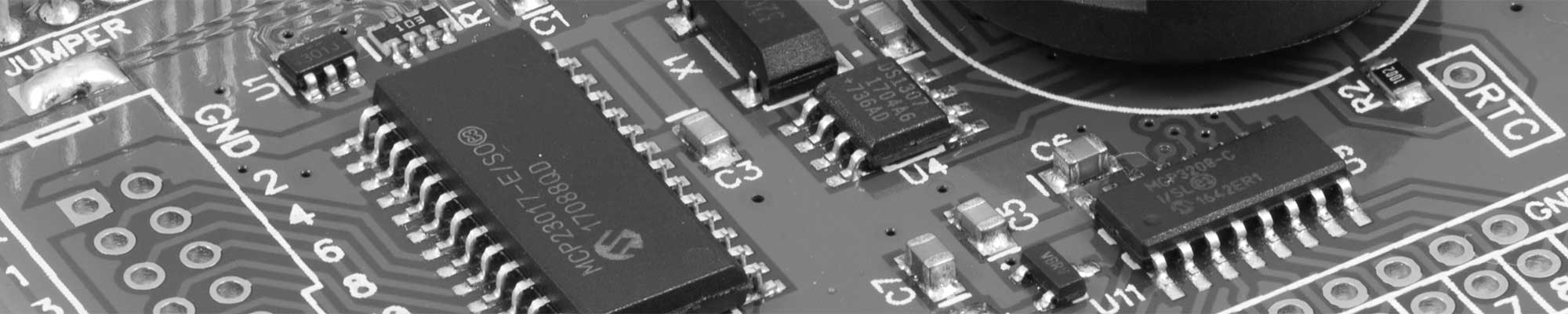# The Blog: Pt1000 thermistor with the ADC Pi V2

12 Sep 2013This post was submitted by Craig Edwards Dagda who has been using a Pt1000 thermistor with the ADC Pi V2 board and was getting unexpected results and explains how he resolved the problems:

Here is what I’m doing.

I wanted to use a Pt1000 thermistor to take temperature readings, sounds simple enough.

I'm thought using an ADCPiV2 would be ideal.  Just put the thermistor as half a potential divider and read off the voltage.  Bit of maths to work out the resistance and convert it into temperature.

Got it all set up, read the voltages, did the math and came out that I’m working in a room at 5'C!

So checked all again and found my problem (first problem, but didn't know this at the time) seemed to be the voltage checked with a multimeter across the ADCPiV2 pins was not quite the same as reported from the Pi.  So read the data sheet for the MCP3424 several times, was getting the right number out, but when converting it to a voltage the error crept in.  So it turned out to be the full scale being defined as 5v, with the tolerance and standard values of the resistors on the front end of the chip it actually comes to between 5.06v and 5.1v depending on the board (i've got 3 of them).  So problem solved and away we go.

Well not quite, it got me a bit closer.

I tested the little board with thermistor on it with power supply and multimeter and all was fine, readings and calculation all fine.

Plug it into the Pi and the voltage across the thermistor was low compared to stand alone.

Played around quite a while with my wiring but couldn't find anything wrong, even went to using 4 wires (like a wheatstone bridge) to make sure the wires' resistance wasn't giving me grief.

Turns out that my original maths was wrong.

V2 * R1 = R2
V1-V2

V1 = supply voltage
V2 = voltage across thermistor
R1 = fixed resistor
R2 = thermistor

Standard PD. Nothing immediately obviously wrong.  Worked ok standalone too.

However it's all in the implementation.

The Pt1000 thermistor has a resistance of 1000ohm at 0'C (about 1080ohm at room temp).

So I put

R1 = 1KOhm, giving me a nice middle of the road output at 0'C (it actually was 1000ohm too! on the dot)

V1 = 4.93V, measured on a meter.
V2 = 2.49V, measured on a meter and on Pi.

Giving me
R2=1020ohm

Thermistor has 385ohm per 100'C so I'm at 5.7'C!!

Where I went wrong was R2 is not the resistance of the thermistor, it's the resistance of the thermistor and the input resistors on the ADCPiV2.

So

1/R2    = 1/Rt + 1/Ri

Rt = thermistor resistance

Ri = input resistance (10k + 6.8k)

1/1020 = 1/Rt + 1/16800

Makes a huge difference

Rt = 1086 ohm which is a more believable 24'C

So the moral of my story is

Buffering is great (luckily the op amp won't need to swing to the supply rails).

Either that or I may swap the input resistors for 1Mohm and 680kohm giving the same operation but 1/1680000 becomes manageable (65>5>1ohm difference in calculated values by increasing resistors by x1, x10 and x100).

Further changes and information:

Trying to calibrate at 100'C does put steam on the circuit board which in turn reduces the resistance so beware!!

Thermistor calibration is pretty close to the spec sheet (occasional ohm here and there) so don't need to change any of the parameters to get a good reading.

Upped the fixed resistor to 2K2 (2170 exact) to give a voltage range that can be sampled directly into the MCP3424 keeping the supply voltage at 5V.

However this will have a problem if the temp goes above 110'C as the voltage will be over the 2.048v reference maximum.  Can't see that happening today unless there is a fire!
Oh and instead of converting the integer reply from the ADC to a voltage, have just used the integers directly instead of the V, it's only used as a ratio anyway. But for direct reading with the 2k2 the V1 of 5v becomes (5/2.048)*maxcount, with maxcount being dependant on the bit resolution. So for 12 bit it is 5000.

The other alternative is get the DELTAV1 and put my own resistors and voltage regulator to have a direct reading into the MCP3424.

Just thought you'd like to know any pitfalls in case anyone else gets in touch.  It would be a beach to diagnose remotely!

We would like to thank Craig for his detailed explanation for the solution to the problem and for allowing us to publish this on our blog.

Back to the Blog.